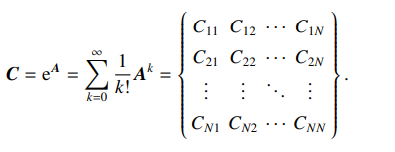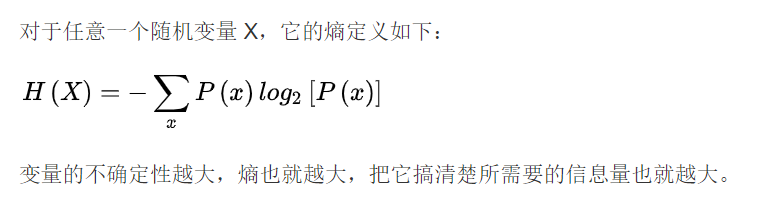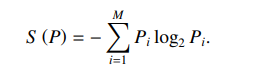## 通信序列熵笔记

• 考虑了节点间的所有可能路径
• 表征任意一对节点间的全局通信能力。• 矩阵中的每个元素都为通信函数
• 表征所有节点间的通信能力

• 通信序列 $$P = {P_1, P_2, P_3, · · · , P_k , · · · , P_M}$$

• 序列P的长度为 $$M = N(N + 1)/2$$

• 序列中每一个元素可表示为 $$P_k=C_{ij}/\sum{(1\leq{k}\leq{M},1\leq{i}\leq{j}\leq{N})}$$ 其中 $$\sum=\sum_{i=1}^N\sum_{j=1}^NC_{ij}$$

$$\sum_{k=1}^MP_k=1$$

• 将通信矩阵的对角线及对角线上方的元素进行分析, 将这些元素进行归一化, 得到通信序列

•• 用来描述不确定事件的不确定程度，是随机变量不确定度的度量。随机变量不确定度越大，熵越大；反之越小。

• 通信序列熵• 将通信序列的香农熵称为网络的通信序列熵

## 参考文献

Built with Hugo
Theme Stack designed by Jimmy